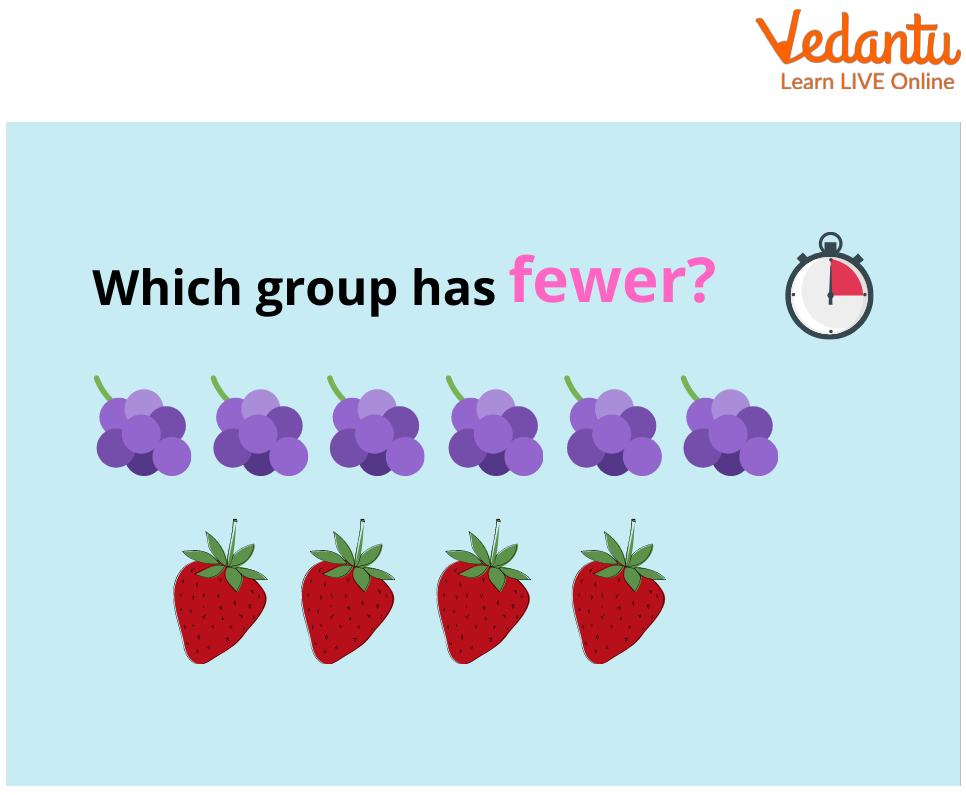Courses
Courses for Kids
Free study material
Free LIVE classes
More

# What Does Fewer Mean in Math?LIVE
Join Vedantu’s FREE Mastercalss

## Meaning of Fewer in Maths

Fewer is the comparative of few; a smaller number.

We often get confused with the two terms viz: some and a few, what difference do they hold? Well, some are something that refers to the uncountable things while fewer refers to people or thighs that are very less in number.

So what is the meaning of fewer than? Well, fewer in maths express that a number or amount is surprisingly large. No fewer than 50 students attended the school yesterday. No fewer than 80 percent of the students marked the correct answer.

On this page, we will understand what fewer means in math along with the list of solved questions to understand the same.

## What Does Fewer Mean in Math: List of Explanations

1. What is three more than a number?

Explanation: The more in "3 more than a number" points to the addition operator. As we are not provided a specific number, we replace it with a variable (such as ‘k’). Hence, "3 more than a number" can be written in an expression form as x + 3.

2. How do you use fewer?

'Fewer' and 'Less'

Explanation: Generally, we use ‘fewer’ when the number of things is countable (fewer apples), while less is used when the things are measurable, not countable, such as "less trouble" or "less time".

3. Are 10 items or less wrong?

Ans: Yes. “10 items or less” is wrong.

Explanation: It should be “10 items or fewer” because “items” are countable. T

## Solved Questions to Understand Fewer in Maths

1. In a basketball game, monkeys scored 14 points fewer than dogs. The dogs scored 99 points. How many scores did monkeys make?

Given: We are given the score of dogs = 99

Need to find: Scored made by monkeys

Solution: Let scores of monkeys be ‘x’

Also, x = 99 - 14

On solving, we get ‘x’ = 85.

Ans: Thus, monkeys scored 85 which is 14 less than the scores of dogs, i.e., 99.

2. Which group of fruits is fewer?Here, we have 6 apples in the first row and 4 watermelons in the second.

We notice that the number of watermelons is fewer than the number of apples. So, the items in the second row are fewer.

From the above text on fewer in math, we understand that fewer is used for small countable things, while less is used for measurable or uncountable objects or items.

Last updated date: 01st Oct 2023
Total views: 141k
Views today: 2.41k

## FAQs on What Does Fewer Mean in Math?

1. Does fewer mean subtract?

Yes, "fewer" means subtraction, however, some questions may confuse you! So, be sure to read the questions carefully!

2. What does 9 fewer mean?

Explanation: 9 less than a number (x) means that 9 is being (subtracted) from that number.

3. What does difference mean in math?

The difference is finding the result by subtracting one number from another. This means that the left of one number when subtracted from another is the difference.

While performing a subtraction, there are three parts:

• The minuend (the number being subtracted from)

• The subtrahend (the number being subtracted), and

• The last is the result or the difference.